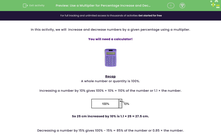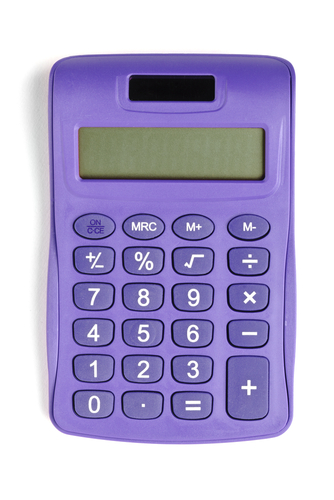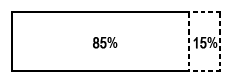# Use a Multiplier for Percentage Increase and Decrease Problems

In this worksheet, students will use a multiplier to increase and decrease numbers by a given percentage. Calculators should be used.Key stage:  KS 3

Curriculum topic:   Number

Curriculum subtopic:   Define, Interpret and Compare Percentages

Difficulty level:#### Worksheet Overview

In this activity, we will  increase and decrease numbers by a given percentage using a multiplier.

You will need a calculator!Recap

A whole number or quantity is 100%.

Increasing a number by 10% gives 100% + 10% = 110% of the number or 1.1 × the number.So 25 cm increased by 10% is 1.1 × 25 = 27.5 cm.

Decreasing a number by 15% gives 100% - 15% = 85% of the number or 0.85 × the number.So 25 cm decreased by 15% is 0.85 × 25 = 21.25 cm.We are going to use this knowledge to answer problems with percentage increases and decreases.

Example

Last year, 30 students attended table tennis club.

This year, the numbers have increased by 30%.

How many students are attending this year?

Method

An increase of 30% is 130% in total = 1.30

We multiply 30 students by 1.30

30 x 1.30 = 39 studentsLet's try some questions like this!

### What is EdPlace?

We're your National Curriculum aligned online education content provider helping each child succeed in English, maths and science from year 1 to GCSE. With an EdPlace account you’ll be able to track and measure progress, helping each child achieve their best. We build confidence and attainment by personalising each child’s learning at a level that suits them.

Get started